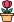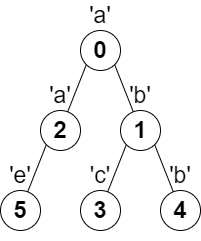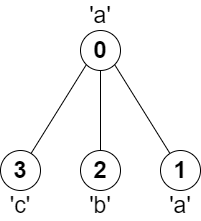엔지니어 게시판
LeetCode 솔루션 분류

# [1/13] 2246. Longest Path With Different Adjacent Characters

### 작성자 정보

•작성
• 작성일

• 104 조회
• 1 댓글
• 0 추천
• 0 비추천

### 본문

2246. Longest Path With Different Adjacent Characters

You are given a tree (i.e. a connected, undirected graph that has no cycles) rooted at node `0` consisting of `n` nodes numbered from `0` to `n - 1`. The tree is represented by a 0-indexed array `parent` of size `n`, where `parent[i]` is the parent of node `i`. Since node `0` is the root, `parent == -1`.

You are also given a string `s` of length `n`, where `s[i]` is the character assigned to node `i`.

Return the length of the longest path in the tree such that no pair of adjacent nodes on the path have the same character assigned to them.

Example 1:```Input: parent = [-1,0,0,1,1,2], s = "abacbe"
Output: 3
Explanation: The longest path where each two adjacent nodes have different characters in the tree is the path: 0 -> 1 -> 3. The length of this path is 3, so 3 is returned.
It can be proven that there is no longer path that satisfies the conditions.
```

Example 2:```Input: parent = [-1,0,0,0], s = "aabc"
Output: 3
Explanation: The longest path where each two adjacent nodes have different characters is the path: 2 -> 0 -> 3. The length of this path is 3, so 3 is returned.
```

Constraints:

• `n == parent.length == s.length`
• `1 <= n <= 105`
• <code style="border: 1px solid rgba(247, 250, 255, 0.12); box-sizing: border-box; --tw-border-spacing-x:0; --tw-border-spacing-y:0; --tw-translate-x:0; --tw-translate-y:0; --tw-rotate:0; --tw-skew-x:0; --tw-skew-y:0; --tw-scale-x:1; --tw-scale-y:1; --tw-pan-x: ;

댓글 1

## 학부유학생님의 댓글

• 익명
• 작성일
``````from collections import defaultdict
class Solution:
def longestPath(self, parent: List[int], s: str) -> int:
graph = defaultdict(list)

for idx, p in enumerate(parent):
if p == -1: continue
graph[p].append(idx)

pathlen = 0

def dfs(curr):
nonlocal pathlen
max1 = max2 = 0
for nxt in graph[curr]:

childlen = dfs(nxt)
if s[nxt] != s[curr]:
if childlen > max1:
max1, max2 = childlen, max1
elif childlen > max2:
max2 = childlen

pathlen = max(pathlen, max1+max2+1)
return max1+1

dfs(0)
return pathlen``````
전체 349 / 1 페이지

• 등록일 02.01
• 등록일 02.01
• 등록일 02.01
• 등록일 02.01
• 등록일 02.01
• 등록일 02.01
• 등록일 02.01
• 등록일 02.01
• 등록일 02.01
• 등록일 02.01
• 등록일 02.01
• 등록일 02.01

• 등록일 01.31
• 등록일 01.31
• 등록일 01.31
• 등록일 01.27
• 등록일 01.27
• 등록일 01.23
• 등록일 01.23

### Stats

• 현재 접속자 55 명
• 오늘 방문자 121 명
• 어제 방문자 1,716 명
• 최대 방문자 2,853 명
• 전체 회원수 535 명